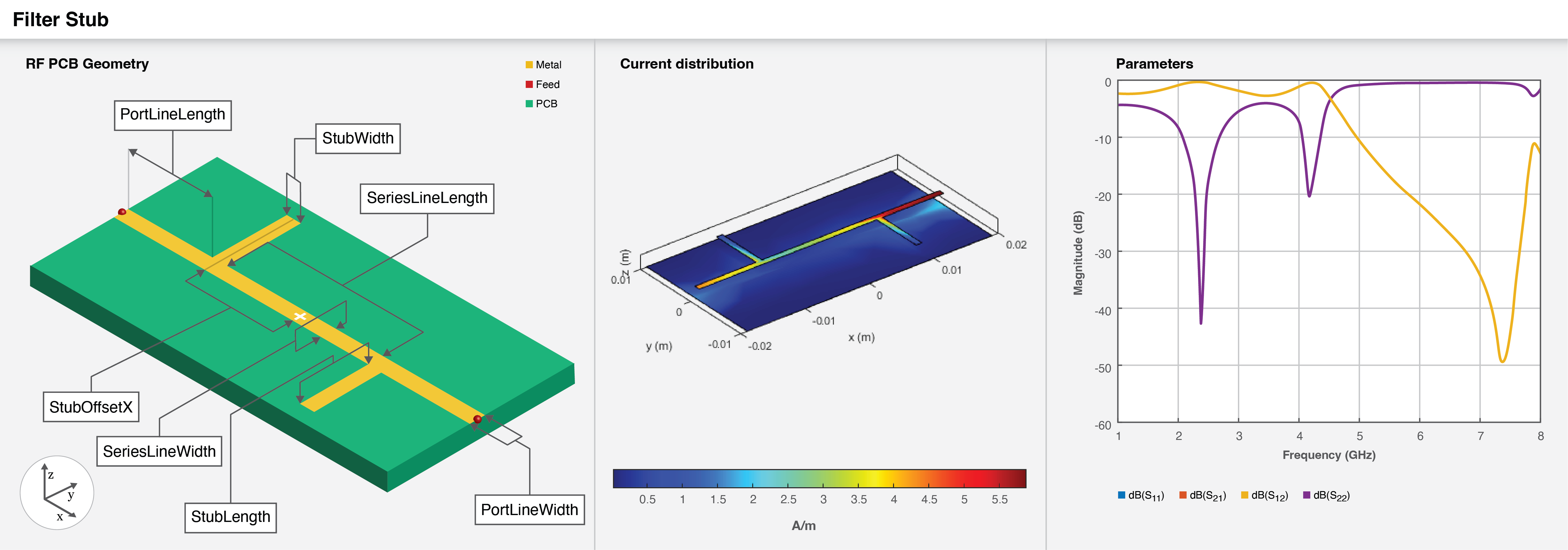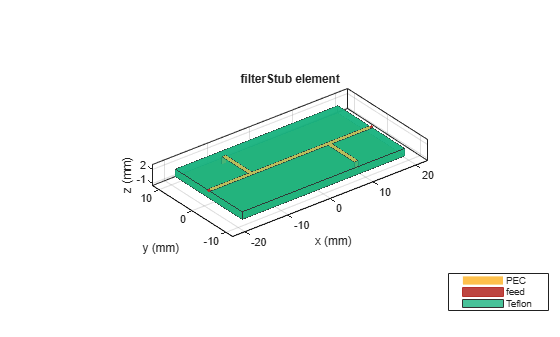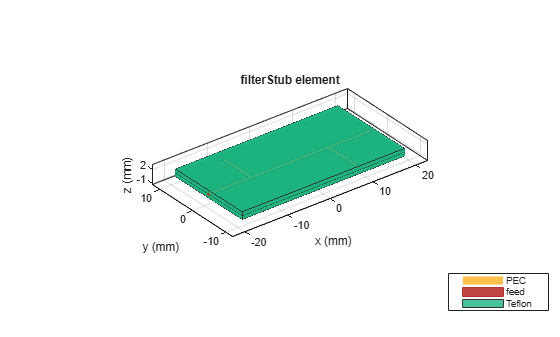# filterStub

Create stub filter in microstrip form

Since R2022b

## Description

Use the `filterStub` object to create a stub filter in the microstrip form.## Creation

### Syntax

``filter = filterStub``
``filter = filterStub(Name=Value)``

### Description

example

````filter = filterStub` creates a default stub with default property values for a passband frequency centered around 2 GHz.```

example

````filter = filterStub(Name=Value)` sets Properties using one or more name-value arguments. For example, `filterStub(PortLineLength=9e-4)` creates a stub filter with a port line length of `9e-4` meters. Properties not specified retain their original values.```

## Properties

expand all

Length of the input and output lines in meters, specified as a positive scalar.

Example: `filter = filterStub(PortLineLength=0.0014)`

Data Types: `double`

Width of the input and output lines in meters, specified as a positive scalar.

Example: `filter = filterStub(PortLineWidth=8e-4)`

Data Types: `double`

Length of the series line in meters, specified as a positive scalar or a two-element vector.

Example: ```filter = filterStub(SeriesLineLength=0.0070)```

Data Types: `double`

Width of the series line in meters, specified as a positive scalar or a two-element vector.

Example: `filter = filterStub(SeriesLineWidth=8e-4)`

Data Types: `double`

Length of the stubs in meters, specified as a positive scalar or a two-element vector.

Example: ```filter = filterStub(StubLength=[0.0078 0.0078])```

Data Types: `double`

Width of the stubs in meters, specified as a positive scalar or a two-element vector.

Example: ```filter = filterStub(StubWidth=[0.0078 0.0078])```

Data Types: `double`

X-offset from the origin in meters, specified as a positive scalar or a two-element vector.

Example: ```filter = filterStub(StubFeedOffsetX=0.0087)```

Data Types: `double`

Direction of the stubs, specified as a two-element vector of:

• `1` — Indicates that the stub points to the top.

• `0` — Indicates that the stub points to the bottom.

Example: `filter = filterStub(StubDirection=[0 1])`

Data Types: `double`

Flag to indicate short-circuited stubs, specified as a two-element vector of:

• `1` — Indicates stub is short-circuited.

• `0` — Indicates stub is open-circuited.

Example: `filter = filterStub(StubShort=[1 1])`

Data Types: `double`

Height of the stub filter from the ground plane in meters, specified as a positive scalar. For multilayer dielectrics, use the `Height` property to create the filter between the two dielectric layers.

Example: `filter = filterStub(Height=0.0028)`

Data Types: `double`

Width of the ground plane in meters, specified as a positive scalar.

Example: ```filter = filterStub(GroundPlaneWidth=0.0048)```

Data Types: `double`

Type of dielectric material used as a substrate, specified as a `dielectric` object. The dielectric material in a `filterStub` object with default properties is Teflon.

Example: `d = dielectric("FR4");` `filter = filterStub(Substrate=d)`

Type of metal used in the conducting layers, specified as a `metal` object. The type of metal in a `filterStub` object with default properties is PEC.

Example: `m = metal("Copper");` `filter = filterStub(Conductor=m)`

## Object Functions

 `charge` Calculate and plot charge distribution `current` Calculate and plot current distribution `feedCurrent` Calculate current at feed port `getZ0` Calculate characteristic impedance of transmission line `layout` Plot all metal layers and board shape `mesh` Change and view mesh properties of metal or dielectric in PCB component `shapes` Extract all metal layer shapes of PCB component `show` Display PCB component structure or PCB shape `sparameters` Calculate S-parameters for RF PCB objects

## Examples

collapse all

Create a short-circuited stub filter.

`filter = filterStub(StubShort=[1 1])`
```filter = filterStub with properties: PortLineLength: 0.0150 PortLineWidth: 9.0000e-04 SeriesLineLength: 0.0080 SeriesLineWidth: 9.0000e-04 StubLength: [0.0083 0.0083] StubWidth: [9.0000e-04 9.0000e-04] StubOffsetX: [-0.0090 0.0090] StubDirection: [1 0] StubShort: [1 1] Height: 0.0016 GroundPlaneWidth: 0.0200 Substrate: [1x1 dielectric] Conductor: [1x1 metal] ```

Visualize the filter.

`show(filter)`Create a multilayered dielectric stub filter.

```filter = filterStub; filter.Substrate = dielectric(Name={'Teflon','Teflon'},EpsilonR=[2.1 2.1], ... LossTangent=[0 0],Thickness=[0.8e-3 0.8e-3]); filter.Height = 0.8e-3;```

Visualize the filter.

`show(filter);`Pozar, David M. Microwave Engineering. 4th ed. Hoboken, NJ: Wiley, 2012.

 Wen-Hua Tu and Kai Chang. “Compact Microstrip Bandstop Filter Using Open Stub and Spurline.” IEEE Microwave and Wireless Components Letters 15, no. 4 (April 2005): 268–70. https://doi.org/10.1109/LMWC.2005.845739.